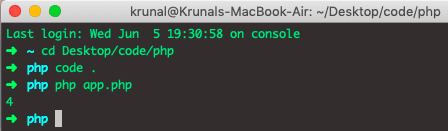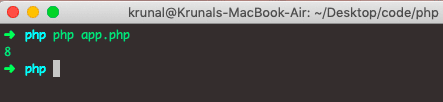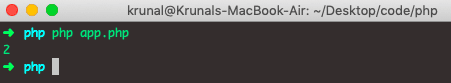# PHP array_count: The Complete Guide

If you have installed SPL, you can hook into count() by implementing the interface Countable. The interface has only one method, Countable::count(), which returns a return value for the count() function.

## PHP array_count

PHP array_count() is a built-in function that returns the number of elements in the array. The array count() function is used to count the items of an array or the properties of an object.

PHP count() function count all elements in an array or something in an object.

### Syntax

See the following syntax.

`count(array,mode);`

### Parameters

The array parameter is required and returns the number of elements in the array.

The mode parameter is Optional. Specifies the mode. Possible values:

1. 0 – Default. It does not count all elements of multidimensional arrays
2. 1 – Counts the array recursively (counts all the elements of multidimensional arrays)

### Example

See the following code example of the PHP count() method.

```<?php

// app.php

\$brands = ['Louis Vuitton', 'Gucci', 'Prada', 'Dior'];
echo count(\$brands);```

See the below output.In the above code, we have four items in the array. So, it will return 4 in the output.

The count() function may return 0 for the variable which is not set, but it may also return 0 for the variable that has been initialized with the empty array.

The isset() function should be used to test whether the variable is set or not.

## Recursive count() in PHP

See the following code.

```<?php

// app.php

\$food = array('fruits' => array('orange', 'banana', 'apple'),
'veggie' => array('carrot', 'collard', 'pea'));

echo count(\$food, COUNT_RECURSIVE);
```

Now run the app.php file and see the output.In the above code, we have passed the COUNT_RECURSIVE parameter, which is why it counts every item in the array and sub-arrays. That is why the total of 8 elements returns 8.

If you do not pass the COUNT_RECURSIVE, it will return 2 elements.

```<?php

// app.php

\$food = array('fruits' => array('orange', 'banana', 'apple'),
'veggie' => array('carrot', 'collard', 'pea'));

echo count(\$food);
```

See the output.If you want to run through the large arrays, then don’t use the count() function in the loops; it’s over the head in performance; copy the count() value into a variable and use that value in loops for better performance.

See the following code example.

```// Bad approach

for(\$i=0;\$i<count(\$arr);\$i++)
{
// calculations
}

// Good approach

\$arr_length = count(\$arr);
for(\$i=0;\$i<\$arr_length;\$i++)
{
// calculations
}```

The following function of one line is recursively to find a number of items that are not arrays.

```function count_elt(\$array, &\$count=0){
foreach(\$array as \$v) if(is_array(\$v)) count_elt(\$v,\$count); else ++\$count;
return \$count;
}```

When it comes to multidimensional arrays, you do not want all levels of the array tree. The following function is beneficial.

```<?php

// \$limit is set to the number of recursions

function count_recursive (\$array, \$limit) {
\$count = 0;
foreach (\$array as \$id => \$_array) {
if (is_array (\$_array) && \$limit > 0) {
\$count += count_recursive (\$_array, \$limit - 1);
} else {
\$count += 1;
}
}
return \$count;
}
?>```

That’s it for this tutorial.

Categories PHP

This site uses Akismet to reduce spam. Learn how your comment data is processed.